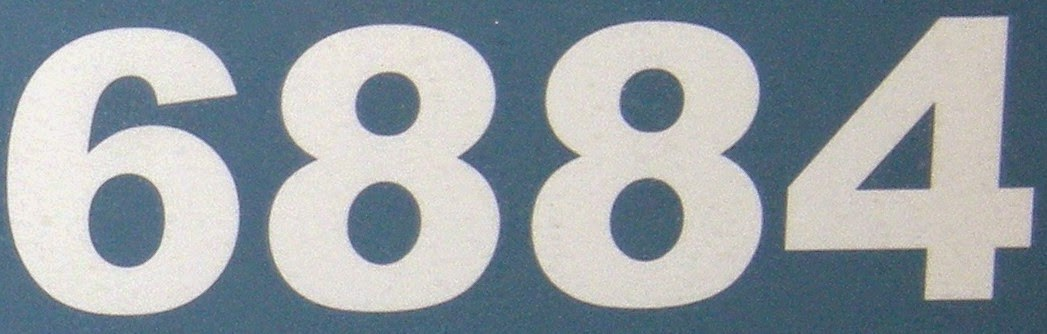Tuesday, December 23, 2014

6884

6884 = 2 x 2 x 1721.

6884 is the number of lines through at least two points of a 7 x 25 grid of points (A160847).

6884 has a representation as a sum of two squares: 6884 = 222 + 802.Source: OEIS